# Quiz : Cases

Plant wide rates: It is a pre-determined overhead rate which is used to allocate the overhead costs to actual units produced. It is calculated using the budgeted overhead and budgeted cost driver. The cost driver usually is the budgeted direct labor hours. Applied overhead: The total overhead allocated to actual production is called the applied overhead. Unit overhead cost: The overhead applied to actual production divided by the number of units produced is the overhead cost per unit. 1.Calculate the pre-determined plant wide overhead rate and the applied overhead for each product using direct labor hours as the cost driver: Calculate the pre-determined overhead rate as shown below: It is calculated by dividing estimated overhead by the expected level of activity (direct labor hours).Therefore, the pre-determined plant wide overhead rate is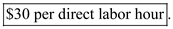Calculate the applied overhead for products deluxe speaker and regular speaker as shown below: It is calculated by applying pre-determined plant wide overhead rate per hour to the direct labor hours spent in producing the product. Overhead applied to deluxe speaker isTherefore, overhead applied to deluxe speaker is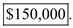Overhead applied to regular speaker isTherefore, overhead applied to regular speaker is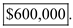2.Calculate the overhead cost per unit of each product: Overhead cost per unit is calculated by dividing the overhead applied to the product by the number of units actually produced. Calculate the overhead cost per unit of deluxe speaker as shown below:Therefore, overhead cost per unit of deluxe speaker is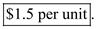Calculate the overhead cost per unit of regular speaker as shown below:Therefore, overhead cost per unit of deluxe speaker is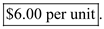Calculate the effect on the profitability if 10,000 hours were used instead of 5,000 hours of deluxe speaker: Calculate the effect on profitability as shown below: In case of additional labor hours spent the profit will decrease to the extent of additional overhead incurred which isTherefore, the additional overhead applied is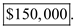Therefore, the profit will decrease to the extent of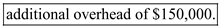Direct tracing: Direct tracing is a process of determining costs associated to direct materials. Costs for whole direct operations are determined in total thereby knowing the profitability of the production concerned with different product lines. Driver tracing: Driver tracing is a process of determining costs associated to any particular cost driver. A cost for each driver is determined separately, thereby knowing the costs associated with each cost driver. Allocation: Allocation refers to the process of assigning costs to each operation and each material concerned with a specific operation in order to determine the total costs and cost per unit of the production. C S Division uses activity based cost accounting system. The major difference between functional based and activity based cost accounting is that under functional approach costs are assigned to each functional division concerned with production. While in case of activity based costing costs are assigned to per activity performed for producing a product.

The differences between the C S Division's products and P Division's products are as under: 1. The P Division is concerned with manufacturing telephones in different segments. Therefore it is dealing in physical production of goods. While C S Division is concerned with telecasting different channels, thus it is providing service to customers. 2. As P Division is associated with physical production, so its products are tangible in nature. While as C S Division is a service industry, hence its production is intangible. 3. The P Division manufactures its products in Midwest and C S Division is providing its cable service in Ohio. 4. Because of high quality and advanced input usage P Division's products are expensive. But C S Division provides service at reasonably low price. 5. A single product is manufactured by P Division that is telephone. But C S Division offers three products to customers, basic channel package, enhanced package and a premium package of 25 plus 2 more channels.

There is no answer for this question

There is no answer for this question

There is no answer for this question

There is no answer for this question

There is no answer for this question

There is no answer for this question